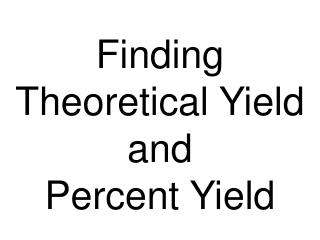DownloadDownload PresentationFinding Theoretical Yield and Percent Yield

# Finding Theoretical Yield and Percent Yield

Télécharger la présentation## Finding Theoretical Yield and Percent Yield

- - - - - - - - - - - - - - - - - - - - - - - - - - - E N D - - - - - - - - - - - - - - - - - - - - - - - - - - -
##### Presentation Transcript

1. Finding Theoretical Yield and Percent Yield

2. Theoretical Yield • The theoretical yield is the maximum amount of product in grams that can be made. • In other words it’s the amount of product possible in grams as calculated through the stoichiometry problem. • This is different from the actual yield, which is the amount one actually produces and measures.

3. Actual Yield Theoretical Yield Percent Yield = x 100 Percent Yield One finds the percent yield by comparing the amount actually obtained (actual yield) to the amount it was possible to make (theoretical yield). • Practice Problems Ch 8 #27, 28, 30

4. Finding Empirical Formulas

5. Calculating Empirical Formulas One can calculate the empirical formula from the percent composition.

6. Calculating Empirical Formulas The compound para-aminobenzoic acid (you may have seen it listed as PABA on your bottle of sunscreen) is composed of carbon (61.31%), hydrogen (5.14%), nitrogen (10.21%), and oxygen (23.33%). Find the empirical formula of PABA.

7. Assuming 100.00 g of para-aminobenzoic acid, C: 61.31 g x = 5.105 mol C H: 5.14 g x = 5.09 mol H N: 10.21 g x = 0.7288 mol N O: 23.33 g x = 1.456 mol O 1 mol 12.01 g 1 mol 14.01 g 1 mol 16.00 g 1 mol 1.01 g Calculating Empirical Formulas

8. C: = 7.005  7 H: = 6.984  7 N: = 1.000 O: = 2.001  2 5.09 mol 0.7288 mol 0.7288 mol 0.7288 mol 5.105 mol 0.7288 mol 1.458 mol 0.7288 mol Calculating Empirical Formulas Calculate the mole ratio by dividing by the smallest number of moles:

9. Calculating Empirical Formulas These are the subscripts for the empirical formula: C7H7NO2

10. Combustion Analysis • Compounds containing C, H and O are routinely analyzed through combustion in a chamber like this. • C is determined from the mass of CO2 produced. • H is determined from the mass of H2O produced. • O is determined by difference after the C and H have been determined.

11. Practice Problem • Analysis of an unknown organic compound containing only C, H, and O shows: A 14.3 g sample of the unknown burns to produce 13.7 g CO2 and 11.2 g H20. • Determine % of each element in original compound. • Determine the empirical formula for original compound. • If the average molecular weight of this unknown compound is 138 g/mole , what is the molecular formula?